Contents

# Contents

## Idea

In type II string theory on orientifolds (Dai-Leigh-Polchinski 89), one says O-plane for the fixed point locus of the $\mathbb{Z}_2$-involution (see at real space).

O-planes carry D-brane charges in KR-theory (Witten 98), see (DMR 13) for a mathematical account. They serve RR-field tadpole cancellation and as such play a key role in the construction of intersecting D-brane models for string phenomenology.

## Properties

### T-Duality with type I string theory

Under T-duality, type I string theory is dual to type II string theory with orientifold planes (reviewed e.g. in Ibanez-Uranga 12, section 5.3.2 - 5.3.4):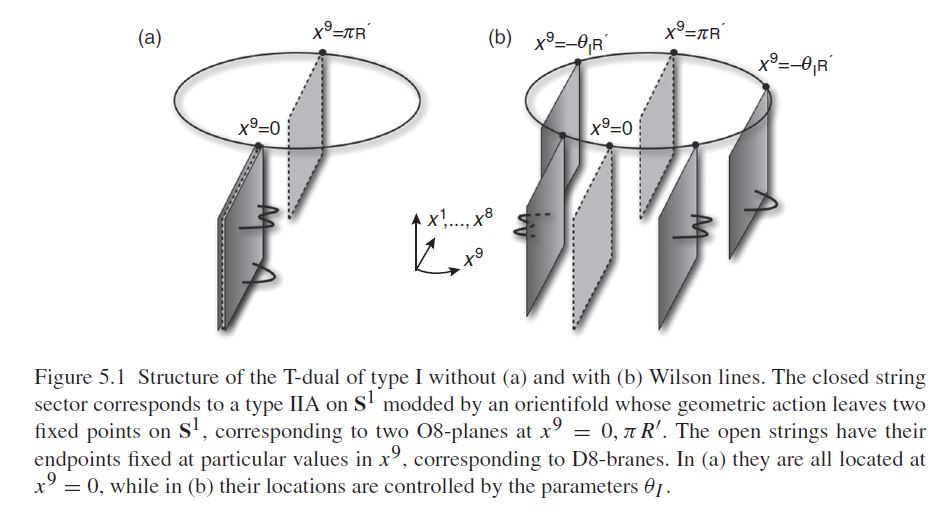### O-Plane charge

O-planes carry effective negative RR-charge which may (must) cancel against the actual RR- D-brane charge via RR-field tadpole cancellation.

#### For flat orientifolds

The charge of the spacetime-filling $O9$-plane of plain type I string theory (type II string theory on the orientifold $\mathbb{R}^{9,1}\sslash \mathbb{Z}_2$ with trival spacetime $\mathbb{Z}_2$-action) is found by worldsheet-computation to be $-32$ in units of D9-brane charge:

(1)$q_{O9^-} \;=\; -32 \, q_{D9}$
###### Remark

counting of D-branes on orientifolds

Beware that there is some convention involved in assigning an absolute value of unit D-brane charge $q_{D9}$. A common choice in the literature is to mean by “one D-brane” one of the two pre-images on the covering space, in which case its obsolute charge is to be

(2)$q_{Dp} \;=\; 1/2$

(e.g. BDHKMMS 01, p. 46-47). From BLT 13, p. 318: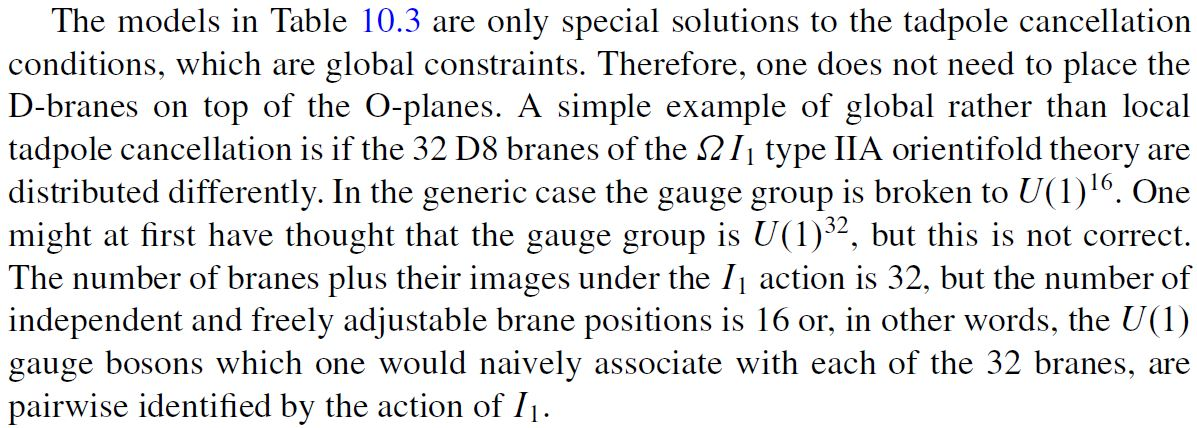This means that RR-field tadpole cancellation here requires the presence of 32 D-branes (or rather, by Remark : 16 and their $\mathbb{Z}_2$-mirror images), hence a space-filling D9-brane with Chan-Paton bundle of rank $32$, corresponding to a gauge group SO(32). For more on this see at type I string theoryTadpole cancellation and SO(32)-GUT.

From this the O$p^-$-brane charge for $p \leq n$ follows from T-duality (as above) with respect to KK-compactification on a d-torus $\mathbb{T}^d$ with $\mathbb{Z}_2$-action given by canonical coordinate reflection

$\array{ \mathbb{Z}_2 \times \mathbb{R}^{10-d-1,1} \times \mathbb{T}^d &\longrightarrow& \mathbb{R}^{10-d-1,1} \times \mathbb{T}^d \\ (\sigma, (\vec x, \vec y)) &\mapsto& (\vec x, - \vec y) } \,.$

This results in $O(9-d)^-$-planes with worldvolume $\mathbb{R}^{10-d-1,1}$. But since the orbifold $\mathbb{T}^d\sslash \mathbb{Z}_2$ now has $2^d$ singularities /fixed points (this Example) there are now $2^d$ such $O(9-d)^-$-planes.

Since the number of D-branes does not change under T-duality, the total O-plane charge should be the same as before

$2^d \cdot q_{O(9-d)} \;=\; 1 \cdot q_{O9} \;=\; -32 \cdot q_{D9} \;=\; - 2^5 \cdot q_{D9}$

which means that the $O(9-d)^-$-plane charge is

$q_{O(9-d)^-} \;=\; - 2^{5-d} \cdot q_{D(p-d)}$

or equivalently

(3)$q_{O p^-} \;=\; - 2^{ p - 4 } \cdot q_{D p}$

In summary, we have the following table of O-plane charges on flat orbifolds:

O-plane
species
charge
$q_{O p^-}/q_{D p}$
transverse
d-torus
fixed points
$\left\vert\left( \mathbb{T}^d\right)^{\mathbb{Z}_2}\right\vert$
$O9^-$$-32$$\mathbb{T}^0$$\phantom{1}1$
$O8^-$$-16$$\mathbb{T}^1$$\phantom{1}2$
$O7^-$$-\phantom{1}8$$\mathbb{T}^2$$\phantom{1}4$
$O6^-$$-\phantom{1}4$$\mathbb{T}^3$$\phantom{1}8$
$O5^-$$-\phantom{1}2$$\mathbb{T}^4$$16$
$O4^-$$-\phantom{1}1$$\mathbb{T}^5$$32$

In particular the O4-plane has negative unit charge (in units of D4-brane charge $q_{D4}$), so that the total charge of $-32$ here comes entirely from the number $32 = 2^5$ of fixed points of the $\mathbb{Z}_2$-action on $\mathbb{T}^5$.

O-plane charges of different dimension may be present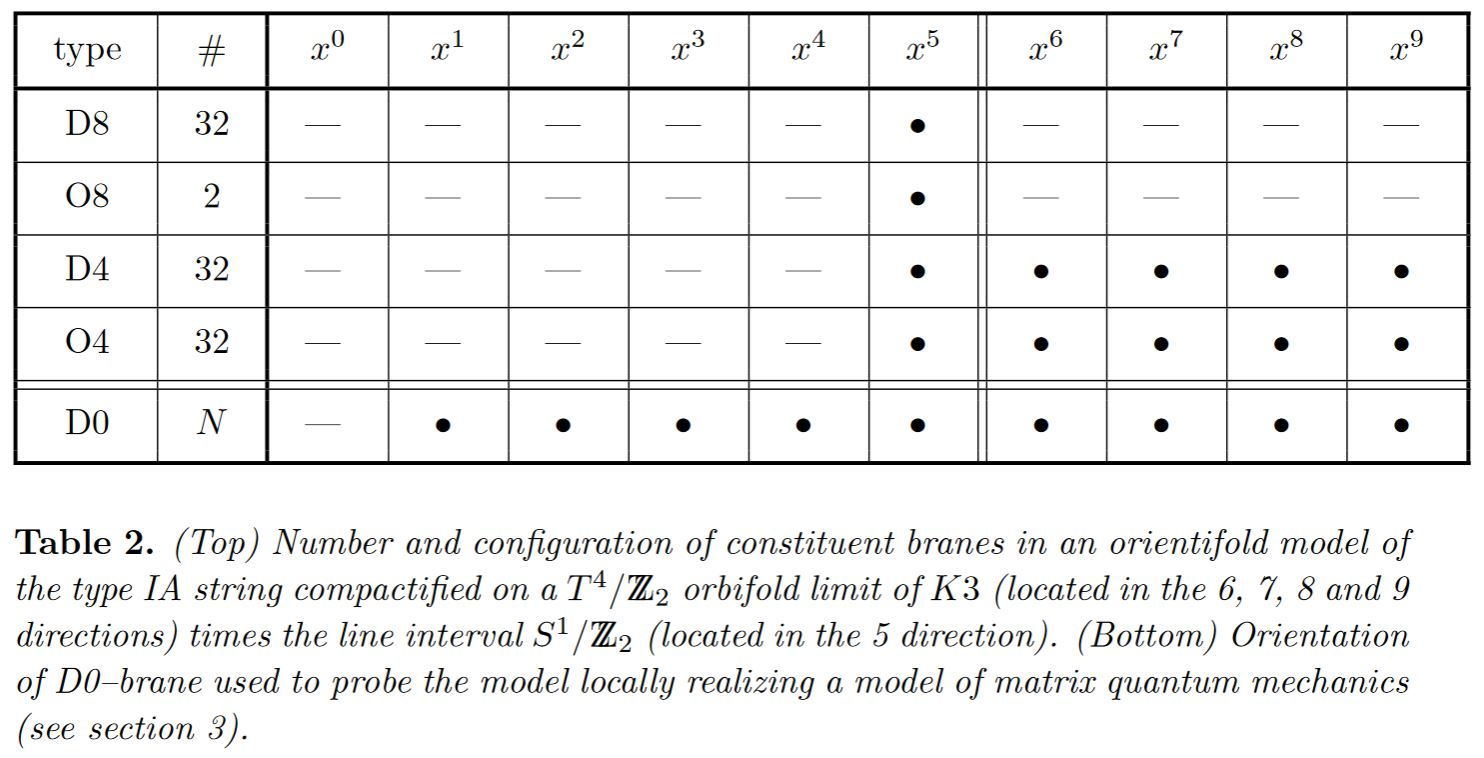graphics grabbed from Johnson 97

#### In the presence of discrete torsion

In the presence of discrete torsion in the B-field and/or the RR-fields, this charge structure of orientifold planes on flat orbifolds gets further modified (Hanany-Kol 00, Sec. 2.1, see Bergman-Gimon-Sugimoto 01, Sec. 1):graphics grabbed from Bergman-Gimon-Sugimoto 01

(In comparing this last table with the above table, notice that this shows the Op-plane charge in units of $q_{Dq} \coloneqq 1/2$ as in (2).)

#### In differential equivariant KR-theory

A proposal for a formalization of a much more general formula for O-plane charge, regarded in differential equivariant KR-theory is briefly in Distler-Freed-Moore 09, p. 6.

### Duality with M-Theory

The possible O-planes in M-theory are $MO1$ ($\leftrightarrow$M-wave), MO5 ($\leftrightarrow$M5-brane) and MO9 (Hanany-Kol 00 around (3.2), HSS 18, Prop. 4.7).

Under the duality between M-theory and type IIA string theory the O8-plane is identified with the MO9 of Horava-Witten theory: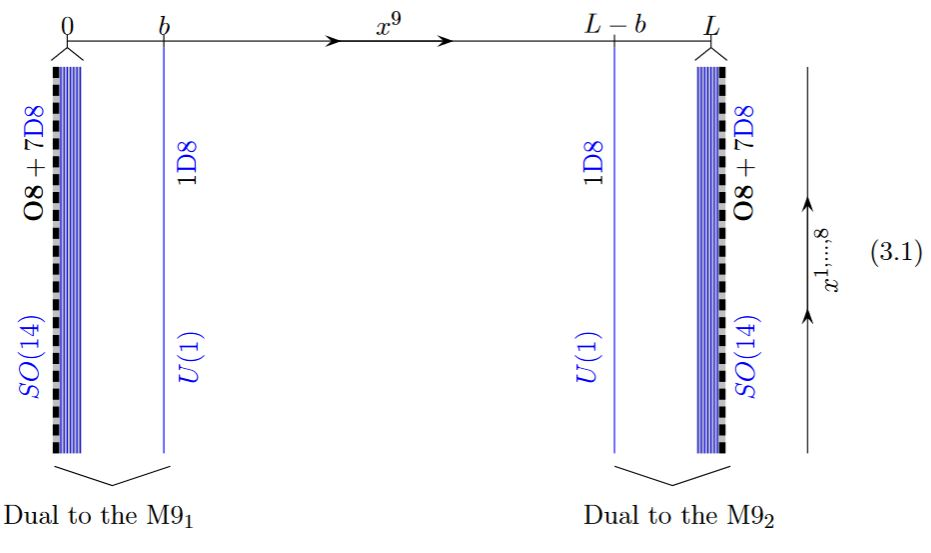graphics grabbed from GKSTY 02, section 3

while the O4-plane is dual to the MO5 (Hori 98, Gimon 98, Sec. III, AKY 98, Sec. II B, Hanany-Kol 00, 3.1.1)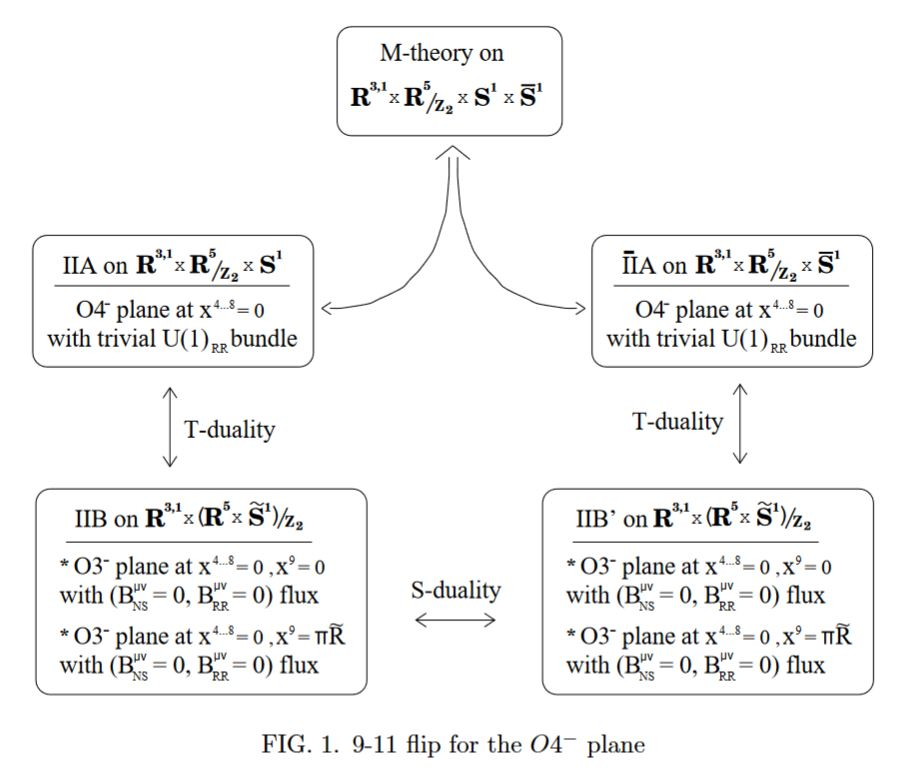graphics grabbed from Gimon 98

and the $O0$ to the MO1 (Hanany-Kol 00 3.3)

### Fractional branes at O-planes

By the discussion at D-branes ending on NS5 branes, a black D6-brane may end on a black NS5-brane, and in fact a priori each brane NS5-brane has to be the junction of two black D6-branes.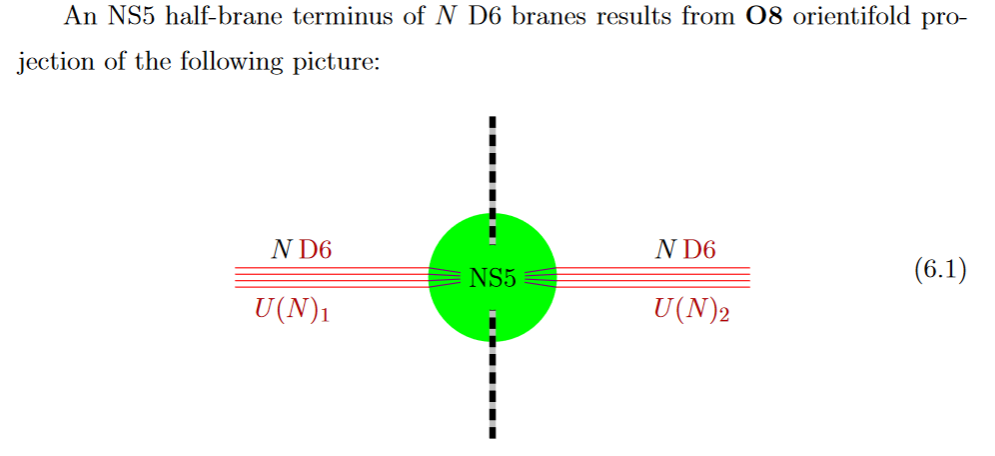from GKSTY 02

If in addition the black NS5-brane sits at an O8-plane, hence at the orientifold fixed point-locus, then in the ordinary $\mathbb{Z}/2$-quotient it appears as a “half-brane” with only one copy of D6-branes ending on it: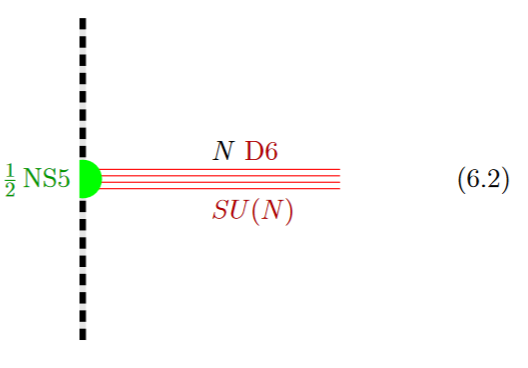from GKSTY 02

(In Hanany-Zaffaroni 99 this is interpreted in terms of the 't Hooft-Polyakov monopole.)

The lift to M-theory of this situation is an M5-brane intersecting an M9-brane: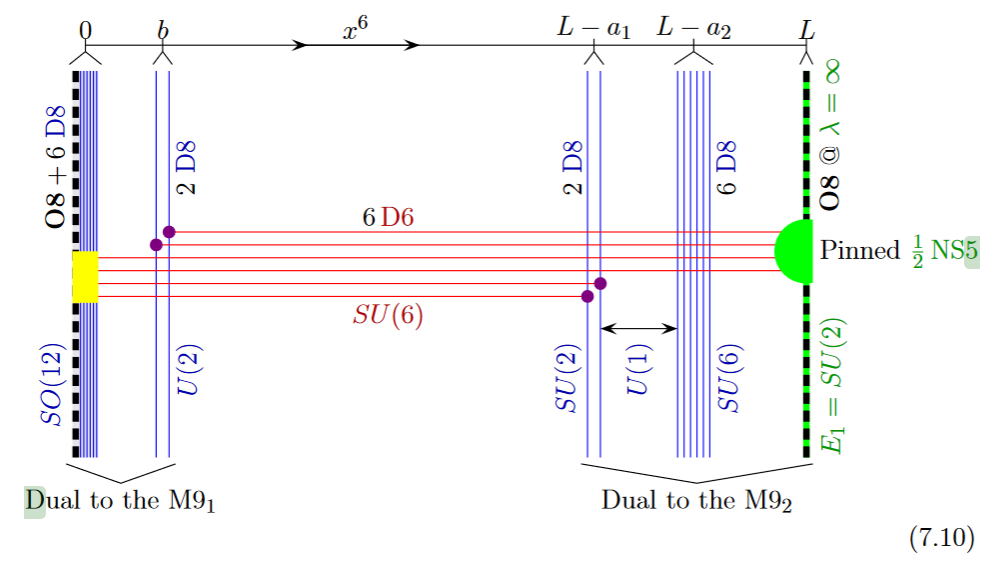from GKSTY 02

Alternatively the O8-plane may intersect the black D6-branes away from the black NS5-brane: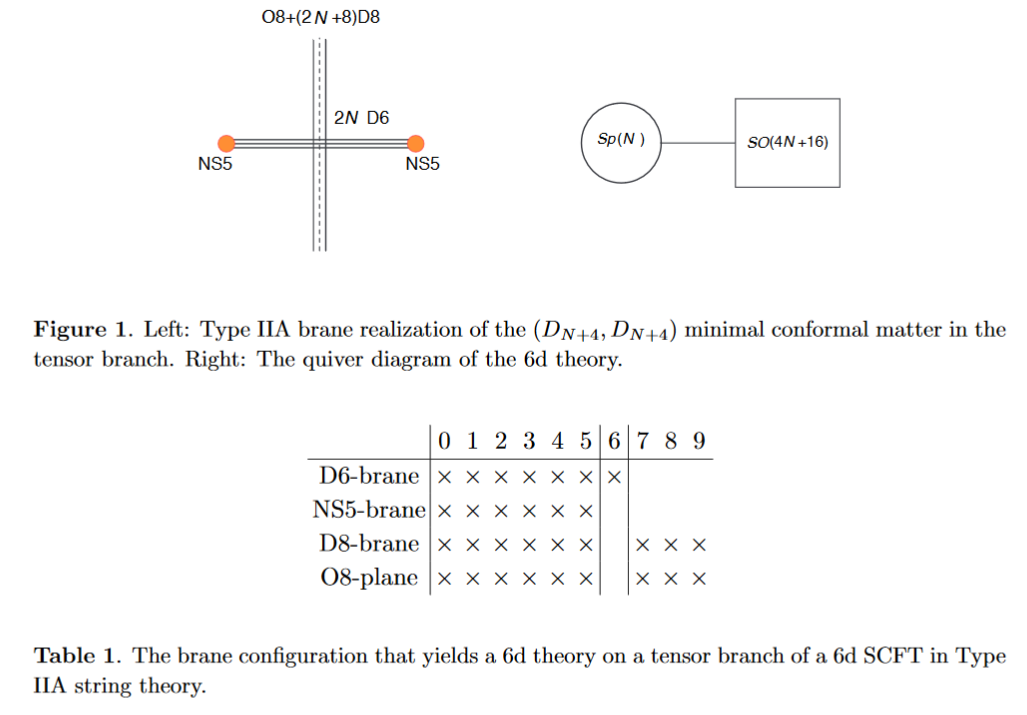from HKLY 15

In general, some of the NS5 sit away from the O8-plane, while some sit on top of it: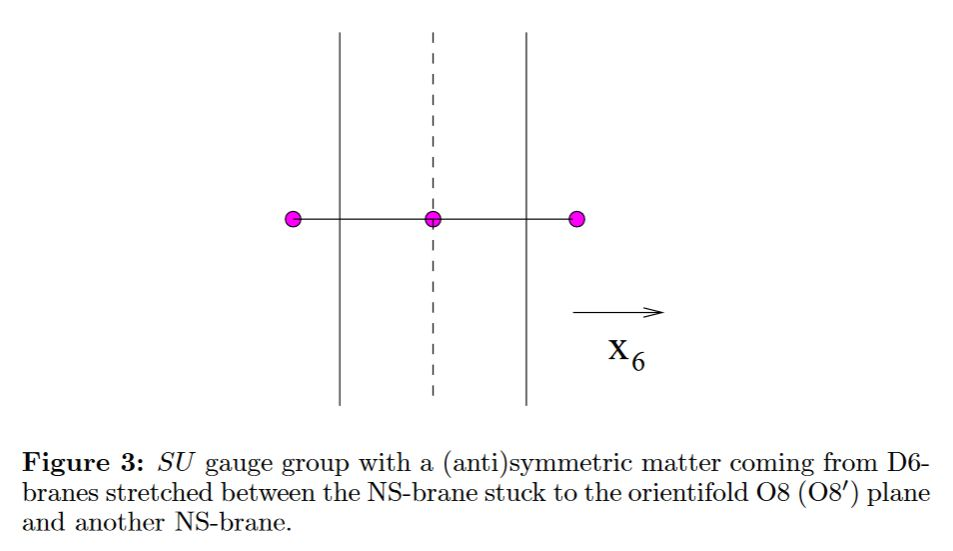See also at intersecting D-brane models the section Intersection of D6s with O8s.

### General

The term “orientifold” originates around

• Jin Dai, R.G. Leigh, Joseph Polchinski, p. 12 of New Connections Between String Theories, Mod.Phys.Lett. A4 (1989) 2073-2083 (spire:25758)

Other early accounts include

Textbook accounts:

### With discrete torsion

O-Plane charge in the presence of discrete torsion:

### In terms of KO-theory

O-Plane charge in differential equivariant KR-theory:

reviewed/surveyed in

Actual construction of twisted differential orthogonal K-theory and its relation to D-brane charge in type I string theory (on orientifolds):

### Examples / Models

The Witten-Sakai-Sugimoto model for QCD on O-planes:

### Lift to M-theory

Lift to M-theory (MO5, MO9):

• Kentaro Hori, Consistency Conditions for Fivebrane in M Theory on $\mathbb{R}^5/\mathbb{Z}_2$ Orbifold, Nucl.Phys.B539:35-78, 1999 (arXiv:hep-th/9805141)

• Eric Gimon, On the M-theory Interpretation of Orientifold Planes (arXiv:hep-th/9806226, spire:472499)

• Changhyun Ahn, Hoil Kim, Hyun Seok Yang, $SO(2N)$ $(0,2)$ SCFT and M Theory on $AdS_7 \times \mathbb{R}P^4$, Phys.Rev. D59 (1999) 106002 (arXiv:hep-th/9808182)

• E. Gorbatov, V.S. Kaplunovsky, J. Sonnenschein, Stefan Theisen, S. Yankielowicz, section 3 of On Heterotic Orbifolds, M Theory and Type I’ Brane Engineering, JHEP 0205:015, 2002 (arXiv:hep-th/0108135)

• Amihay Hanany, Barak Kol, On Orientifolds, Discrete Torsion, Branes and M Theory, JHEP 0006 (2000) 013 (arXiv:hep-th/0003025)

• Hirotaka Hayashi, Sung-Soo Kim, Kimyeong Lee, Futoshi Yagi, 6d SCFTs, 5d Dualities and Tao Web Diagrams (arXiv:1509.03300)

The intersection with (p,q)5-brane webs:

• Hirotaka Hayashi, Sung-Soo Kim, Kimyeong Lee, Masato Taki, Futoshi Yagi, More on 5d descriptions of 6d SCFTs, JHEP10 (2016) 126 (arXiv:1512.08239)

• Amihay Hanany, Alberto Zaffaroni, Issues on Orientifolds: On the brane construction of gauge theories with $SO(2n)$ global symmetry, JHEP 9907 (1999) 009 (arXiv:hep-th/9903242)

• Gabi Zafrir, Brane webs in the presence of an $O5^-$-plane and 4d class S theories of type D, JHEP07 (2016) 035 (arXiv:1602.00130)